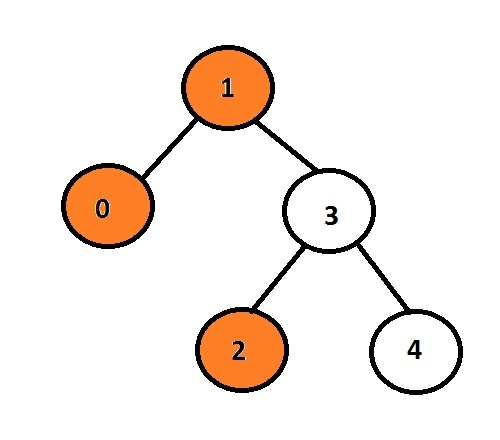# Print Left View of a Binary Tree in C language

The task is to print the left nodes of a given binary tree. Firstly user will insert data hence generating binary tree and than print left view of the tree so formed.

Every node can have at most 2 child so here the program must traverse only the left pointer associated with a node

If left pointer is not null means it will have some data or pointer associated with it if not than it will be the left child to be printed and displayed as an output.

## Example

Input : 1 0 3 2 4
Output : 1 0 2here, orange nodes represent left view of a binary tree.

In the given figure node with data 1 is a root node so it will be printed than going to left child it will print 0 and than it will goto 3 and print its left child which is 2.

We can use recursive approach for storing the level of node and repeatedly shifting to the other.

The below code shows the c implementation of the algorithm given

## Algorithm

START
Step 1 -> create node variable of type structure
Declare int data
Declare pointer of type node using *left, *right
Step 2 -> create function for inserting node with parameter as new_data
Declare temp variable of node using malloc
Set temp->data = new_data
Set temp->left = temp->right = NULL
return temp
Step 3 -> declare function void left_view(struct node* root, int level, int* highest_level)
IF root = NULL
Exit
End
IF *highest_level < level
Print root->data
Set *highest_level = level
End
Recursively call left_view(root->left, level + 1, highest_level)
Recursively call left_view(root->right, level + 1, highest_level)
Step 4 -> Declare Function void left(struct node* root)
Set int highest_level = 0
Call left_view(root, 1, &highest_level)
Step 5-> In main()
Call New passing value user want to insert as struct node* root = New(1)
Call left(root)
STOP

## Example

#include <stdio.h>
#include <stdlib.h>
//create a structure of a node
struct node {
int data;
struct node *left, *right; //this pointer will point to the nodes attached with a node
};
struct node* New(int new_data) {
struct node* temp = (struct node*)malloc(sizeof(struct node));
//allocating memory to a pointer    dynamically
temp->data = new_data;
temp->left = temp->right = NULL;
return temp;
}
void left_view(struct node* root, int level, int* highest_level) {
if (root == NULL) //if there is no node that means no data
return;
// this function will retrun the root node if there is only root node in a tree
if (*highest_level < level) {
printf("%d\t", root->data);
*highest_level = level;
}
// Recursive function
left_view(root->left, level + 1, highest_level);
left_view(root->right, level + 1, highest_level);
}
void left(struct node* root) {
int highest_level = 0;
left_view(root, 1, &highest_level);
}
int main() {
printf("left view of a binary tree is : ");
struct node* root = New(1);
root->left = New(0);
root->right = New(3);
root->right->left = New(2);
root->right->right = New(4);
left(root);
return 0;
}

## Output

If we run above program then it will generate following output.

left view of a binary tree is : 1 0 2

Updated on: 22-Aug-2019

232 Views